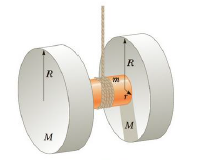Chapter 8, Problem 40P

Chapter
Section
Textbook Problem

An oversized yo-yo is made from two identical solid disks each of mass M = 2.00 kg and radius R = 10.0 cm. The two disks are joined by a solid cylinder of radius r = 4.00 cm and mass m = 1.00 kg as in Figure P8.40. Take the center of the cylinder as the axis of the system, with positive torques directed to the left along this axis. All torques and angular variables are to be calculated around this axis. Light string is wrapped around the cylinder, and the system is then allowed to drop from rest, (a) What is the moment of inertia of the system? Give a symbolic answer, (b) What torque does gravity exert on the system with respect to the given axis? (c) Take downward as the negative coordinate direction. As depicted in Figure P8.40, is the torque exerted by the tension positive or negative? Is the angular acceleration positive or negative? What about the translational acceleration? (d) Write an equation for the angular acceleration a in terms of the translational acceleration a and radius r (Watch the sign!) (e) Write Newton’s second law for the system in terms of m, M, a, T, and g. (f) Write Newton’s second law for rotation in terms of I, α, T, and r. (g) Eliminate α from the rotational second law with the expression found in part (d) and find a symbolic expression for the acceleration a in terms of m, M, g, r, and R. (h) What is the numeric value for the system’s acceleration? (i) What is the tension in the string? (j) How long does it take the system to drop 1.00 m from rest?Figure P8.40

(a)

To determine

The moment of inertia of the system.

Explanation

Given info:

Formula to calculate the moment of inertia of the disc is,

Id=MR22

• Id is the moment of inertia of the disk,
• M is the mass of the disk,
• R is the radius of the disk.

Formula to calculate the moment of inertia of the cylinder is,

Ic=mr22

• m is the mass of the cylinder,
• r is the radius of the cylinder,
• Ic is the moment of inertia of the cylinder,

Formula to calculate the moment of inertia of the system,

I=2Id+Ic

• I is the moment of inertia of the system,

Use M

(b)

To determine

The torque exerted by the gravity on the system.

(c)

To determine

The torque exerted by the tension is either positive or negative. The angular acceleration is either positive or negative and The Translational acceleration is either positive or negative.

(d)

To determine

The equation for the angular acceleration in terms of translational acceleration.

(e)

To determine

The Newton second law in terms of m, M, a, T and g.

(f)

To determine

The Newton second law for rotation in terms of I, a, T and g.

(g)

To determine

The acceleration a in terms of m, M, g, r and R.

(h)

To determine

The numerical value for the system’s acceleration a.

(i)

To determine

The Tension in the string T.

(j)

To determine

The time to drop the system 1.00m from rest.

Still sussing out bartleby?

Check out a sample textbook solution.

See a sample solution

The Solution to Your Study Problems

Bartleby provides explanations to thousands of textbook problems written by our experts, many with advanced degrees!

Get Started

Show that 1 cubic meter contains 1000 L.

An Introduction to Physical Science

Why does a stars life expectancy depend on its mass?

Horizons: Exploring the Universe (MindTap Course List)

What is the structure and function of the cytoskeleton?

Biology: The Dynamic Science (MindTap Course List)

The diaphragm is a ______ muscle. a. smooth b. skeletal c. dome-shaped d. a and c

Biology: The Unity and Diversity of Life (MindTap Course List)